• 协方差矩阵matlab
2021-04-26 19:05:36

matlab求协方差矩阵

>> A=[0,0,0;2,0,2;]

A =

0 0 0

2 0 2

>> v = diag(cov(A))'

v =

2 0 2

help里面的cov 函数，你自己看一下吧!

MATLAB 怎么计算协方差

>> x=rand(1,5);

>> y=2*rand(1,5);

>> cov(x,y) %计算协方差

ans =

0.1079 -0.0225

-0.0225 0.6148

协方差分析是建立在方差分析和回归分析基础之上的一种统计分析方法。 方差分析是从质量因子的角度探讨因素不同水平对实验指标影响的差异。一般说来，质量因子是可以人为控制的。 回归分析是从数量因子的角度出发，通过建立回归方程来研究实验指标与一个(或几个)因子之间的数量关系。但大多数情况下，数量因子是不可以人为加以控制的。

为什么考虑风险时，与市场组合的协方差更重要?

组合的方差是有协方差矩阵的和决定的

假如有N个资产构成一个组合

那么协方差有N的平方减N个

而方差只有N个

所以前者重要

这个告诉我们 股票的收益率看的是它对投资组合的风险贡献率 而不是自己的波动

- -说得比较抽象

Excel、Matlab的算出的协方差矩阵为什么不同

大概是因为两种用的公式不一样。 因为你不可能根据样本得到协方差矩阵， 而只能根据样本做一个估计， 既然是估计那么当然有多种公式都是可行的， 而不能说哪一种就一定错

【本文标题和网址】股票的协方差矩阵-matlab求协方差矩阵：http://www.jjta.cn/pzzx/160113.html

声明本站分享的文章旨在促进信息交流，不以盈利为目的，本文观点与本站立场无关，不承担任何责任。如有伤害到您的利益，请联系站长删除！

更多相关内容
• [今天看论文的时候又看到了协方差矩阵这个破东西，以前看模式分类的时候就特困扰，没想到现在还是搞不清楚，索性开始查协方差矩阵的资料，恶补之后决定马上记录下来，嘿嘿~协方差矩阵协方差也只能处理二维问题，那维...

[今天看论文的时候又看到了协方差矩阵这个破东西，以前看模式分类的时候就特困扰，没想到现在还是搞不清楚，索性开始查协方差矩阵的资料，恶补之后决定马上记录下来，嘿嘿~

协方差矩阵

协方差也只能处理二维问题，那维数多了自然就需要计算多个协方差，比如n维的数据集就需要计算 n!(n−2)!∗2 个协方差，那自然而然我们会想到使用矩阵来组织这些数据。给出协方差矩阵的定义：

Cn∗n=(ci,j,ci,j=cov(Dimi,Dimj))

这个定义还是很容易理解的，我们可以举一个三维的例子，假设数据集有三个维度，则协方差矩阵为：[协方差定义       在概率论和统计学中，协方差用于衡量两个变量的总体误差。而方差是协方差的一种特殊情况，即当两个变量是相同的情况。       期望值分别为E(X) = μ 与

必须要明确一点，协方差矩阵计算的是不同维度之间的协方差，而不是不同样本之间的。以下的演示将使用Matlab，为了说明计算原理，不直接调用Matlab的cov函数：

首先，随机生成一个10*3维的整数矩阵作为样本集，10为样本的个数，3为样本的维数。

>> mySample=fix(rand(10,3)*50)

mySample =

40 7 32

45 48 1

6 47 42

45 24 46

31 40 33

4 7 37

13 21 37

27 45 19

47 39 32

48 47 8

根据公式，计算协方差需要计算均值，前面特别强调了，协方差矩阵是计算不同维度之间的协方差，要时刻牢记这一点。样本矩阵的每行是一个样本，每列是一个维度，因此我们要按列计算均值。为了描述方便，我们先将三个维度的数据分别赋值：

>> dim1=mySample(:,1)

dim1 =

40

45

6

45

31

4

13

27

47

48

>> dim2=mySample(:,2)

dim2 =

7

48

47

24

40

7

21

45

39

47

>> dim3=mySample(:,3)

dim3 =

32

1

42

46

33

37

37

19

32

8

计算dim1与dim2，dim1与dim3，dim2与dim3的协方差：

>> cov12=sum((dim1-mean(dim1)).*(dim2-mean(dim2)))/(size(mySample,1)-1)

cov12 =

78

>> cov13=sum((dim1-mean(dim1)).*(dim3-mean(dim3)))/(size(mySample,1)-1)

cov13 =

-120.2444

>> cov13=sum((dim1-mean(dim1)).*(dim3-mean(dim3)))/(size(mySample,1)-1)

cov13 =

-120.2444

>> cov13=sum((dim1-mean(dim1)).*(dim3-mean(dim3)))/(size(mySample,1)-1)

cov13 =

-120.2444

>> cov23=sum((dim2-mean(dim2)).*(dim3-mean(dim3)))/(size(mySample,1)-1)

cov23 =

-126.9444

协方差矩阵的对角线上的元素就是各个维度的方差，下面我们依次计算这些方差：

>> var1=std(dim1)^2

var1 =

301.1556

>> var2=std(dim2)^2

var2 =

268.9444

>> var3=std(dim3)^2

var3 =

216.0111

这样，我们就得到了计算协方差矩阵所需要的所有数据，可以调用Matlab的cov函数直接得到协方差矩阵：

>> cov(mySample) ans = 301.1556 78.0000 -120.2444 78.0000 268.9444 -126.9444 -120.2444 -126.9444 216.0111

计算的结果，和之前的数据填入矩阵后的结果完全相同。[转自http://blog.csdn.net/ybdesire/article/details/6270328/协方差的定义 对于一般的分布，直接代入E(X)之类的就可以计算出来了，但真给你一个具体数值的分布，要计算协

展开全文• Dr.Can在他的教学视频（【卡尔曼滤波器】2_数学基础_数据融合_协方差矩阵_状态空间方程_观测器问题）中使用了足球运动员的数据介绍了协方差矩阵的概念和计算方法，原始数据如下图，那么协方差矩阵到底是什么？...

Dr.Can在他的教学视频（【卡尔曼滤波器】2_数学基础_数据融合_协方差矩阵_状态空间方程_观测器问题）中使用了足球运动员的数据介绍了协方差矩阵的概念和计算方法，原始数据如下图，那么协方差矩阵到底是什么？他有什么用？我们怎么计算协方差矩阵？如何编写程序让计算机帮助我们计算协方差矩阵呢？协方差矩阵其实是用来描述一个数据集中不同字段（属性）数据之间的关联程度的。百度词条的解释是：

协方差（Covariance）在概率论和统计学中用于衡量两个变量的总体误差。而方差是协方差的一种特殊情况，即当两个变量是相同的情况。
协方差表示的是两个变量的总体的误差，这与只表示一个变量误差的方差不同。如果两个变量的变化趋势一致，也就是说如果其中一个大于自身的期望值，另外一个也大于自身的期望值，那么两个变量之间的协方差就是正值。如果两个变量的变化趋势相反，即其中一个大于自身的期望值，另外一个却小于自身的期望值，那么两个变量之间的协方差就是负值。

用数学语言，协方差的公式可以表示为：我们用简单的人话来说，就是如果两个字段（属性）正相关的（也就是变化趋势一致），协方差是正的；如果负相关（也就是变化趋势相反），那么协方差就是负的。将协方差和两个属性的标准差相除，我们还可以得到一个区间为（-1,1）的相关系数，用来归一化的表示两个字段（属性）的相关程度：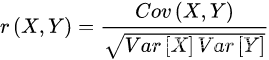DR.CAN提到了一种基于过度矩阵的计算方法，也就是将协方差的计算公式变形一下，这样我们就可以使用matlab中自带的矩阵运算函数得到非常简洁的表达，并且能够高效快速的得到计算结果，计算过程如下：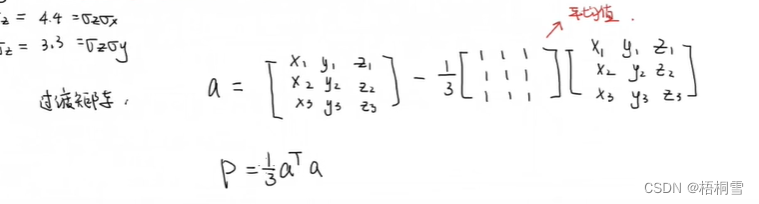我把这种算法写成了matlab函数如下：

function [P] = covarianceMatrix(M)
%输入数据表M矩阵，输出这组数据的协方差矩阵
%   通过引入过度矩阵来简化运算的步骤
N = size(M,1);
dim = size(M,2);
A = ones(N,N);
a = M - 1/N*A*M;
P = 1/N*a'*a
end


我们输入数据调用一下这个函数如下：

M = [
179,74,33;
187,80,31;
175,71,28;
170,72,33;
185,81,32;
177,75,28;
178,71,30;
187,83,35;
190,94,27;
178,73,21;
170,68,29;
183,73,23;
184,78,31;
180,75,24;
180,76,20;
]
covarianceMatrix(M)


运行结果如下：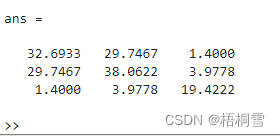我们看到，和Dr.Can用excel计算的结果是一致的。

展开全文matlab
• WEIGHTEDCOV 返回加权协方差的对称矩阵 C，该矩阵根据输入 T×N 矩阵 Y 计算，该矩阵 Y 的行是观察值，列是变量，以及输入 T×1 的观察值权重向量 w。 如果观察结果并非完全相同并且需要根据某些理论假设或知识进行...matlab
• 介绍了Matlab 中如何使用 mean 、var 、std、cov 函数等

今天想用 Matlab 来算一个矩阵数据的均值和方差给我整迷了，好气！！所以做点笔记，以免下次在跳进坑里。

## 1.预备知识

关于算方差等，matlab提供了好几个函数：mean、var、std、cov，首先我们先看看这几个函数咋用的。
以下用到的矩阵都为：

X = [1 2 3; 3 3 6; 4 6 8; 4 7 7; 8 5 9]
=============================
X =

1     2     3
3     3     6
4     6     8
4     7     7
8     5     9


### 1.mean

/*这个函数还好理解，算平均值
如果参数是向量就直接是平均值
如果参数是矩阵，就会有俩种模式
1 返回一个行向量，计算各列的平均值（默认）
2 返回一个列向量，计算各行的平均值*/
>> mean(X(:,1))

ans =

4

>> mean(X(1,:))

ans =

2

>> mean(X)

ans =

4.0000    4.6000    6.6000

>> mean(X,2)

ans =

2.0000
4.0000
6.0000
6.0000
7.3333


如果一个矩阵中有nan的话，如何来求这个矩阵的均值呢？

AAt = reshape(AA, size(AA,1)*size(AA,2),1);
mean(AAt,'omitnan');


### 2.var

这个是用来算方差的，但是分母为（n-1），好像是为了搞成无偏估计，概率论的知识，忘了（😓）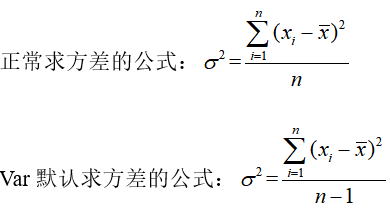var(X,W,DIM)
/*这个函数求方差，公式参上，默认：var(X,0,1)
参数X为向量时：w=0:n-1  w=1:n
参数X为矩阵时：w=0:n-1  w=1:n
dim=1 返回一个行向量，计算各列的方差（默认）
dim=2 返回一个列向量，计算各行的方差
*/
>> var(X(1,:))

ans =

1

>> var(X(:,1))

ans =

6.5000

>> var(X,1,1)

ans =

5.2000    3.4400    4.2400

>> var(X,1,2)

ans =

0.6667
2.0000
2.6667
2.0000
2.8889

>> var(X,0,1)

ans =

6.5000    4.3000    5.3000

>> var(X,0,2)

ans =

1.0000
3.0000
4.0000
3.0000
4.3333


### 3.std

这个函数用来求标准差，就是var开方，调用形式和var类似都是var(X,W,DIM) 这里就不在赘述。

### 4.cov

我们先来回顾一下协方差矩阵是咋算的：
设X为一个向量，其均值表示为E(X)，那么它的方差为：D(X)=E((E(X)-Xi)^2)

这时，又有另一个向量Y(与X长度相同) ,其均值表示为E(Y)
那么它俩的协方差为：cov(X,Y)=E((E(X)-Xi)*(E(Y)-Yi)) = E(XY)－E(X)*E(Y)

协方差与方差有如下关系：
D(X+Y)=D(X)+D(Y)+2Cov(X，Y)
D(X-Y)=D(X)+D(Y)-2Cov(X，Y)

ok，接下来我们一起用matlab 来算算。

cov(X,Y,w)
/*用来计算向量X和Y 的协方差矩阵
没有Y时，用来算X的方差。
w=0:n-1  w=1:n  0 默认
当X是矩阵时：
算的是将每一列当做一个X(或Y),
X若为mxn 的矩阵，得到的是nxn的协方差矩阵*/
>> cov(X,1)

ans =

5.2000    2.2000    4.2000
2.2000    3.4400    2.8400
4.2000    2.8400    4.2400

>> cov(X,0)

ans =

6.5000    2.7500    5.2500
2.7500    4.3000    3.5500
5.2500    3.5500    5.3000

>> cov(X(:,1),X(:,2))

ans =

6.5000    2.7500
2.7500    4.3000


ok，至此已经介绍完了，下面的内容是我自己的思想斗争，大家可以忽略，忽略…

### 5.一个奇怪的东西

我现在突然意识到是我的指导思想错了，我写这篇博客的目的是为了得到一组遥感影像数据（某一类）的协方差矩阵，可现在发现一个cov 完全可以解决，之前想的是：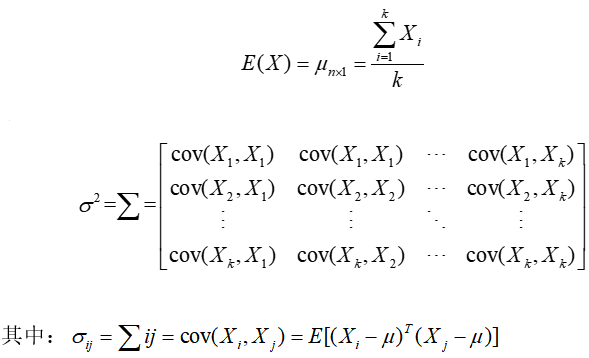其中n是n个波段，k是k个像素点，也就是说，每个像素点有n个灰度；也即有一组数据，有n个维度，这组数据共有k个。之后想求其协方差矩阵。
均值肯定是这k个样本点的均值，为一个n维的向量

从一维向量（行向量1xk）求方差 合理外延就会想到上述公式，但是这个并不是我所需要的，这个是一个kxk的矩阵，我想要的是一个nxn的矩阵，这两个东西到底是何含义，想到爆也想不出来（看来需要时间的积淀了！）

还是看个实例比较容易理解一点。
下面的实例是用来解决上面那个公式引申出来的。

## 2.实例

1.我们先建个矩阵

X = [1 2 3; 3 3 6; 4 6 8; 4 7 7; 8 5 9]
=============================
X =

1     2     3
3     3     6
4     6     8
4     7     7
8     5     9


如上，这是一个5行乘3列的矩阵，我们假设这个矩阵每一列代表遥感影像一个位置处的5个波段的灰度值，也就是说，有个遥感影像，他有三个点，5波段。我们想算他的均值和协方差矩阵，期望得到的均值是一个5行1列的列向量，希望得到的协方差矩阵是一个3行3列实际上是5x5）的矩阵。

我们还想得到一种协方差矩阵，它是5行5列的矩阵。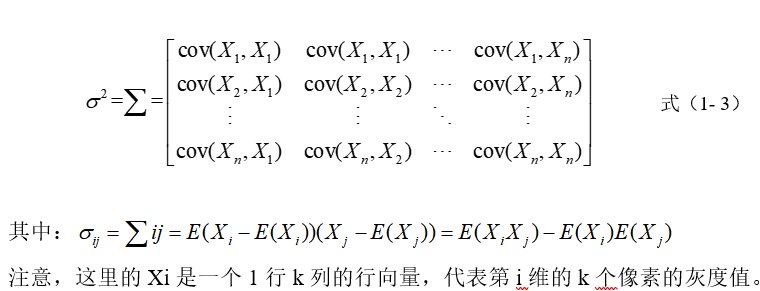2.为了验证，matlab 计算的正确性，我们先手动算一遍：
均值向量为：

mean=
2.0000
4.0000
6.0000
6.0000
7.3333


协方差矩阵1为：

cov=
10.4444     -2.5556     -7.8889
-2.5556      7.4444     -4.8889
-7.8889     -4.8889     12.7778


协方差矩阵2为：

cov=
10.4444     -2.5556     -7.8889
-2.5556      7.4444     -4.8889
-7.8889     -4.8889     12.7778


3.我们来看看用 matlab 自带的函数mean、cov算出来的都是啥：

>> mean(X,1)

ans =

4.0000    4.6000    6.6000

>> mean(X,2)%很遗憾，只有这一个达到了我们的期望

ans =

2.0000
4.0000
6.0000
6.0000
7.3333

>> cov(X)

ans =

6.5000    2.7500    5.2500
2.7500    4.3000    3.5500
5.2500    3.5500    5.3000


那我们将X转置一下在计算会不会好点呢？

>> X=X'

X =

1     3     4     4     8
2     3     6     7     5
3     6     8     7     9

>> mean(X,1)

ans =

2.0000    4.0000    6.0000    6.0000    7.3333

>> mean(X,2)

ans =

4.0000
4.6000
6.6000

>> cov(X)

ans =

1.0000    1.5000    2.0000    1.5000    0.5000
1.5000    3.0000    3.0000    1.5000    2.5000
2.0000    3.0000    4.0000    3.0000    1.0000
1.5000    1.5000    3.0000    3.0000   -1.0000
0.5000    2.5000    1.0000   -1.0000    4.3333


结果还是不符合我们的期望。这是为什么呢？原来这个Matlab计算协方差矩阵的时候，是用每一列作为一个元素（这与我们的期望相符）对角线的方差是计算这一列中的方差（就是其他的元素不考虑，算出这一列中的几个数的均值，之后在算这几个数的方差），非对角线咋算呢？非对角线列是相应列的协方差。
实在想不清楚，可以看看这篇文章：

https://blog.csdn.net/clam_clam/article/details/7335588

## 3.解决方案

OK，到目前为止，我们已经知道指望不上Matlab 自带的函数了（均值可以求，方差不行），那么我们就自食其力，自己写了个函数，用来计算上面想得到的方差。

%mycov.m  by@GHL
%此函数用来计算方差，
%m是一个n行，k列的矩阵
%每一个元素是m中的列向量，有n维
%m中共有k个元素
%最后得到的协方差矩阵是一个k行k列的矩阵
function sigma = mycov(m)%计算
X=mean(m,2);%均值
n=size(m,1);%n维
k=size(m,2);%k个元素
sigma=zeros(k);
for i = 1:k
for j = 1:k
sigma(i,j)=(m(:,i)-X)'*(m(:,j)-X);
end
end
return;
end


验证：

>> X=X'

X =

1     2     3
3     3     6
4     6     8
4     7     7
8     5     9

>> mycov(X)

ans =

10.4444   -2.5556   -7.8889
-2.5556    7.4444   -4.8889
-7.8889   -4.8889   12.7778


## 参考/引用 文章

 clam_clam-CSDN博主：https://blog.csdn.net/clam_clam/article/details/7335588


2020/6/16
【小感慨】
这么一个小玩意花了我一下午来捋清，好气！！差点爆粗口，真是用matlab 的基础还很不好，不管怎么说，这次终于搞清楚了（只差哪两个协方差矩阵没搞明白），希望下次不会在迷了😞😞😞

展开全文matlab 协方差
• end end c = 10.3333 -4.1667 3.0000 -4.1667 2.3333 -1.5000 3.0000 -1.5000 1.0000 c为求得的协方差矩阵，在matlab以矩阵a的每一列为变量，对应的每一行为样本。这样在矩阵a中就有3个列变量分别为a（:,1), a(:,2)...
• 最近在论文里看到很多协方差矩阵的表示方式为 Rx=E{XXT} ，但是我们经常用的都时 cov(X)来计算协方差。 其中：X为一维随机序列或者信号的采样， XT 代表 X 的转置序列 设：x=[10 20 32 44 5 7 2 56 7 2]'; 注意有...Matlab 协方差矩阵
• MATLAB输入矩阵X是m*n大小。请注意： m是#features，n是#samples。 计算的主要代码是： xc = x - sum(x,1)./m; % Remove mean c = (xc' * xc) ./ denom; denom为m-1（m>1）或m(m = 1)。非常有意思的是： a.先...matlab 机器学习
• %% Parametric % 计算 99% 与 95% 水平的...(预期收益率、预期协方差矩阵、样本 个数、收益率间隔、模拟次数) simulated......Matlab 协方差实战上面涉及的内容都比较容易,协方差矩阵似乎也很简单,但实战起来就很...
• 但是在有些用到协方差的算法中， 分母使用了N，而不是N-1。但是由于样本很多，差别不大。 >> v=magic(3) v = 8 1 6 3 5 7 4 9 2 >> cov(v) ans = 7 -8 1 -8 16 -8 ...协方差 协方差矩阵
• 一、源码 function [ COVMAT ] = covarianceMatrix( ...% 这是一个计算协方差矩阵的函数 % inputData 输入数据 % 每一行为一个维度 % 每一列为一个样本 %获得输入数据维度 [m,n] = size(inputData); %创建协...协方差矩阵 MATLAB
• ## 协方差矩阵及matlab实现

万次阅读 多人点赞 2017-09-26 10:26:02
这样，我们就得到了计算协方差矩阵所需要的所有数据，可以调用Matlab的cov函数直接得到协方差矩阵： 图 5 使用Matlab的cov函数直接计算样本的协方差矩阵 计算的结果，和之前的数据填入矩阵后的结果...
• ## matlab协方差矩阵

千次阅读 2018-05-29 15:56:28
参考自文章：http://blog.163.com/yuyang_tech/blog/static/216050083201382610424490/cov(X,Y) 求矩阵X与Y的协方差矩阵。若X大小为M*N，Y为K*P，则X，Y的大小必须满足M*N=K*P，即X，Y的元素个数相同。此时，cov(X,...协方差矩阵 matlab
• 当提供均值和协方差时，此函数绘制二维多变量高斯图。 它不使用 for 循环。 例如：绘图平均值= [10; 11]，cov = [6 0; 0 6] 2D多元高斯函数>> mvg([10;11],[6 0;0 6])matlab
• 今天回顾了之前看到的协方差和协方差矩阵，发现真的缺漏了很多，所以做如下记录。协方差学过概率统计的应该都知道协方差的定义，接下来就简单说明一下。协方差表示的是两个变量的总体的误差，这与只表示一个变量误差...
• 对于协方差矩阵的概念，我只想说，该来的总是要来，躲得了今天跑不了明天。所以今天在此想通过这边文章彻底搞清楚这个梗！（纯手工，有什么不妥的地方，望大家的指点与谅解！） 说起协方差矩阵，自然就会想到均值，...
• 我在协调高斯混合的一些基本理论结果和命令的输出方面遇到了问题...第一次发行A其特征是均值和方差协方差矩阵等于muA=[-1.4 3.2 -1.9]; %mean vectorrhoA=-0.5; %correlation among components in AsigmaA=[1 rh...
• 这样，我们就得到了计算协方差矩阵所需要的所有数据，可以调用Matlab的cov函数直接得到协方差矩阵： 图 5 使用Matlab的cov函数直接计算样本的协方差矩阵 计算的结果，和之前的数据填入矩阵后的结果完全相同。
• 分析由一维到二维数据的方差和协方差与转换矩阵之间的关系，并matlab做图演示
• 协方差矩阵 是一个矩阵，其每个元素是各个向量元素之间的 协方差 。（Wiki） 协方差矩阵的计算以列向量为单位，是列向量各元素之间的关系的表达。 定义为： 一个列向量也叫做一个样本向量，列向量中...
• 由于许多人（大多数是学生）需要以toeplitz矩阵形式的自协方差，因此此函数可以以矩阵形式快速生成输出。 如果需要自相关，则可以简单地用函数内部的xcorr替换xcov命令。matlab
• 返回给定数据矩阵 X 的协方差矩阵（行 = 对象，列 = 特征） % @作者：Kardi Teknomo % http://people.revoledu.com/kardi/index.html 在...Mahalanobis 距离计算的用法matlab
• MATLAB 函数是一种算法，旨在改进 Wishart 分布的协方差矩阵的特征值估计并根据特征值重新计算改进的协方差矩阵。 该函数是 Avishai Ben-David 和 Charles E. Davidson 开发和发布的程序的实现，“来自有限样本...matlab
• 自协方差函数的计算机实现,得益于计算机对矩阵并行运算的优化.我们可以采用数组化编程思想,利用矩阵运算,轻松且快速的计算出自协方差矩阵.matlab
• 从总的多元数据矩阵和显着性水平来看，同质性协方差矩阵通过 Box 的 M 进行测试，给定卡方或 F 近似值作为组样本大小的函数。matlab
• 该函数返回估计的协方差矩阵\ texttt {C_est}以及与此估计的协方差相关的成本函数。 2-比较所有合成数据算法的主要脚本是\ texttt {CompareEst.m}。 3-比较用于LDA / QDA应用程序的估算方法的主要脚本是\ texttt {ML...
• 本博文源于matlab基础，主要对协方差矩阵如何生成进行讲解。该函数使用randn cov使用 randn(m,n) % 生成一个m*n标准正态分布 矩阵 cov(m)% 求出m的协方差矩阵 例子：求出5*6阶服从正态分布随机数的协方差矩阵 >&...matlab
• 计算数据矩阵的 ZCA 白化并返回白化数据和白化/去白化变换矩阵matlab
• matlab中已知协方差矩阵怎样算相关系数？已知协方差矩阵，计算相关系数可以按图中的公式进行。 R就是相关系数矩阵，C为协方差矩阵。 >> a=rand(5,5) a = 0.9501 0.7621 0.6154 0.4057 0.0579 0.2311 0.4565 0.......